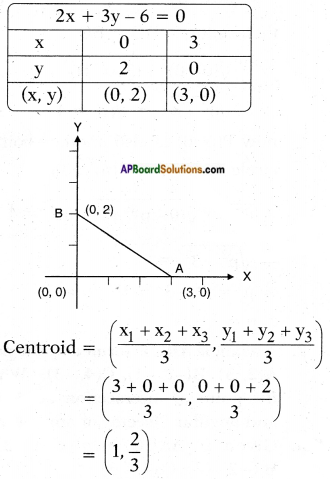## AP State Syllabus SSC 10th Class Maths Solutions 7th Lesson Coordinate Geometry Optional Exercise

AP State Board Syllabus AP SSC 10th Class Maths Textbook Solutions Chapter 7 Coordinate Geometry Optional Exercise Textbook Questions and Answers.

### 10th Class Maths 7th Lesson Coordinate Geometry Optional Exercise Textbook Questions and Answers

Question 1.
Centre of the circle Q is on the Y-axis. And the circle passes through the points (0, 7) and (0, -1). Circle intersects the positive X-axis at (p, 0). What is the value of ‘p’ ?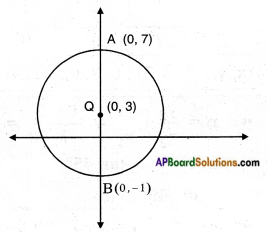Given points A, B are on y – axis.
(∵ their x – coordinate is zero) then they will be the end points (0, 7) and (0, -1).
Centre (Q) = $$\left(\frac{0+0}{2}, \frac{7-1}{2}\right)$$ = (0, 3)
then radius = AQ = BQ
= $$\sqrt{(0-0)^{2}+(3-(-1))^{2}}$$
= $$\sqrt{0^{2}+4^{2}}$$ = √16 = 4
now P(p, 0) is also another point on circle then $$\overline{\mathrm{QP}}$$ is also radius
∴ $$\overline{\mathrm{QP}}$$ = $$\sqrt{(0-p)^{2}+(3-0)^{2}}$$ = 4
⇒ $$\sqrt{p^{2}+3^{2}}$$ = 4
⇒ p2 = 42 – 32 = 16 – 9 = 7
∴ P = ± √7 .Question 2.
A triangle ABC is formed by the points A(2, 3), B(-2, -3), C(4, -3). What is the point of intersection of side BC and angular bisector of angle A?
Given: △ABC, where A (2, 3), B (- 2, – 3), C (4, – 3).
Let AD be the bisector to ∠A meeting BC at D.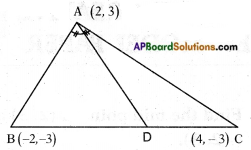Then BD : DC = AB : AC
[∵ The bisector of vertical angle of triangle divides the base in the ratio of other two sides.]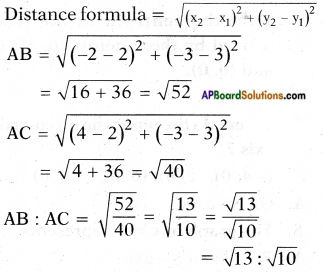Now D is a point which divides $$\overline{\mathrm{BC}}$$ in the ratio √l3 : √10 internally section formula (x, y) =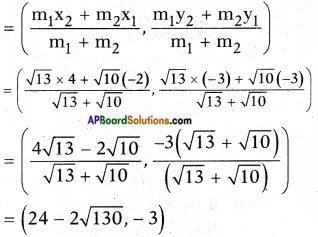(By rationalising the denominator of the x-coordinate).Question 3.
The side BC of an equilateral △ABC is parallel to X – axis. Find the slopes of line along sides BC, CA and AB.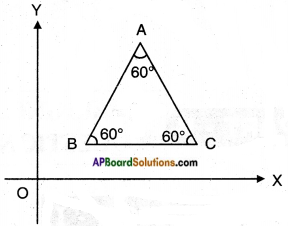Given: △ABC; BC // X – axis.
Slope of BC = tan θ
where θ is the angle made of BC with + ve X – axis.
= tan ‘0’ = ‘0’
Slope of AB = tan 60° = √3
[∵ Each angle of an equilateral triangle is 60°]
Slope of AC = tan 60° = √3

Question 4.
A right triangle has sides ‘a’ and ‘b’ where a > b. If the right angle is bisected then find the distance between ortho centres of the smaller triangles using coordinate geometry.
Given : △ABC; ∠B = 90°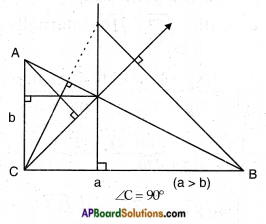Question 5.
Find the centroid of the triangle formed by the line 2x + 3y – 6 = 0 with the coordinate axes.